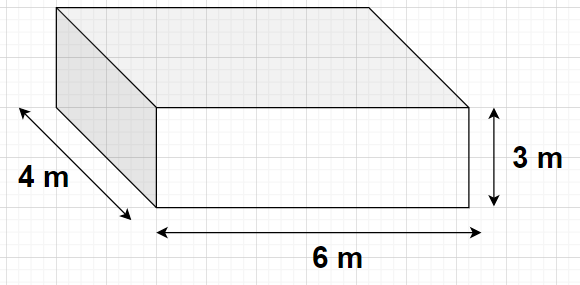QUESTION

# Find the volume of a cuboid measuring 6 m by 4 m by 3 m.Hint- Here, we will proceed by following the general nomenclature of the dimensions of any cuboid and then in order to obtain the volume of the cuboid we will use the formula i.e., Volume of the cuboid = (Length of the cuboid)$\times$(Breadth of the cuboid)$\times$(Height of the cuboid).

Complete step-by-step solution -
Given, the dimensions of the given cuboid are 6 m, 4 m and 3 m
Length of the cuboid = 6 m
Breadth of the cuboid = 4 m
Height of the cuboid = 3 m
As we know that according to the formula, the volume of the cuboid is given by
Volume of the cuboid = (Length of the cuboid)$\times$(Breadth of the cuboid)$\times$(Height of the cuboid)
$\Rightarrow$Volume of the given cuboid = $6 \times 4 \times 3 = 72{\text{ }}{{\text{m}}^3}$
Therefore, the volume of the cuboid with the given dimensions is 72 cubic metre.

Note- In these types of problems, it is very important to verify that the units of all the dimensions (i.e., length, breadth and height) of any cuboid are the same. If all the units are not the same, then we will convert all the units the same by using some unit conversion formulas like 1 m = 100 cm or 1 m = 1000 mm or any other.